### Electrochemistry Class 12th Chemistry Part I CBSE Solution

##### Question 1.Arrange the following metals in the order in which they displace each other from the solution of their salts.Al, Cu, Fe, Mg, and Zn.Answer:The order in which the given metals displace each other from the solution of their salts is given by,Mg>Al> Zn> Fe> CuExplanation -A metal of stronger reducing power displaces another metal of weaker reducing power from its solution of salt. The order of increasing the reducing power of given metals is Cu< Fe< Zn< Al<Mg. Hence, we can interpret as Mg can displace Cu from its salt solution, but Cu cannot displace Mg. The order in which the given metals displace each other from the solution of their salts is given by, Mg>Al> Zn> Fe> Cu. This is hence arranged in decreasing order of its reactivity.Question 2.Given the standard electrode potentials,K+ /K = –2.93V, Ag+ /Ag = 0.80V,Hg2+ /Hg = 0.79VMg2+ /Mg = –2.37 V, Cr3+ /Cr = – 0.74VArrange these metals in their increasing order of reducing power.Answer:Reducing power of metals increase with the decrease of reduction potential. Hence, the increasing order of reducing power will be as,Ag < Hg < Cr < Mg < KExplanation -When the reduction potential is lower, the element has more tendency to get oxidized and thus more will be reducing power. The metal that has more negative electrode potential will be the one with more reducing power. Thus, here potassium(K) has the highest reducing power among the given elements.Question 3.Depict the galvanic cell in which the reactionZn(s) + 2Ag+ (aq) → Zn2+ (aq) + 2Ag(s) takes place. Further show:1) Which of the electrode is negatively charged?2) The carriers of the current in the cell.3) Individual reaction at each electrode.Answer:The galvanic cell corresponding to the given redox reaction can be represented as:Zn|Zn2 +(aq)||Ag +(aq)|Ag1) Zn electrode (anode) is negatively charged because, at this electrode, Zn is oxidized to Zn2+, causing electron accumulation at the anode.2) Electrons (ions) are the carriers of the current in the cell and in the external circuit, current flows from Ag (cathode) to Zn(anode) which is normally opposite to the electron flow which is from anode to cathode.3) At anode:Zn(s)⇒ Zn2 +(aq) + 2e–At cathode:Ag +(aq) + e –⇒ Ag(s)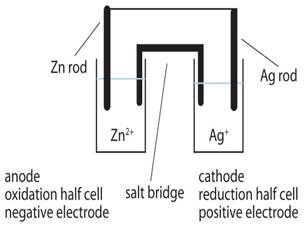Question 4.Calculate the standard cell potentials of galvanic cell in which the following reactions take place:(i) 2Cr(s) + 3Cd2+ (aq) → 2Cr3+ (aq) + 3Cd(ii) Fe2 + (aq) + Ag+ (aq) → Fe3+ (aq) + Ag(s)Calculate the ΔrG0 and equilibrium constant(K) of the reactions.Answer:(i) Known - E0Cr3 +/Cr = - 0.74 VE0Cd2 +/Cd = - 0.40 V∆rG0 = ?K = ?The galvanic cell of the given reaction is written as -Cr(s)|Cr3 +(aq)|| Cd2 +(aq)|Cd(s)→ Reaction 1Hence, the standard cell potential is given as,E0 = E0R - E0L= - 0.40 - (- 0.74)∴ E0 = + 0.34 VTo calculate the standard Gibb’s free energy, ∆rG0, we use,∆rG0 = - nE0F → Equation 1where nF is the amount of charge passed and E0 is the standard reduction electrode potential.Substituting n = 6 (no. of e - involved in the reaction 1), F = 96487 C mol-1,E0 = + 0.34 V in Equation 1, we get,∆rG0 = - 6×0.34V×96487 C mol-1= - 196833.48 CV mol-1= - 196833.48 J mol-1∴ ∆rG0 = - 196.83348 kJ mol-1To find out the equilibrium constant, K, we use the formula,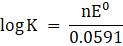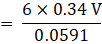log K = 34.5177K = antilog 34.5177∴ K = 3.294 × 1034Thestandard Gibb’s free energy, ∆rG0 is - 196.83348 kJ mol – 1 and equilibrium constant, K is3.294 × 1034(ii) Known -E0Fe3 +/Fe2 + = 0.77VE0Ag+/Ag = 0.80 V∆rG0 = ?K = ?The galvanic cell of the given reaction is written as -Fe2 +(aq)|Fe3 +(aq)|| Ag +(aq)|Ag(s)→ Reaction 1Hence, the standard cell potential is given as,E0 = E0R - E0L= 0.80 - (0.77)∴ E0 = + 0.03 VTo calculate the standard Gibb’s free energy, ∆rG0, we use,∆rG0 = - nE0F → Equation 1where nF is the amount of charge passed and E0 is the standard reduction electrode potential.Substituting n = 1 (no. of e - involved in the reaction 1), F = 96487 C mol-1, E0 = + 0.03V in Equation 1, we get,∆rG0 = - 1×0.03V×96487 C mol-1= - 2894.61 CV mol - 1= - 2894.61 J mol-1∴ ∆rG0 = - 2.89461 kJ mol-1To find out the equilibrium constant, K, we use the formula,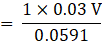log K = 0.5076K = Antilog 0.5076∴ K = 3.218Thestandard Gibb’s free energy, ∆rG0 is - 2.89461 kJ mol – 1 and equilibrium constant, K is3.218Question 5.Write the Nernst equation and emf of the following cells at 298 K:(i) Mg(s)|Mg2+ (0.001M)||Cu2+ (0.0001 M)|Cu(s)(ii) Fe(s)|Fe2+ (0.001M)||H+ (1M)|H2(g)(1bar)| Pt(s)(iii) Sn(s)|Sn2+ (0.050 M)||H+ (0.020 M)|H2(g) (1 bar)|Pt(s)(iv) Pt(s)|Br2(l)|Br–(0.010 M)||H+ (0.030 M)| H2(g) (1 bar)|Pt(s).Answer:Ecell = ?(i) Mg + Cu2 + → Mg2 + + Cu (n = 2)E0Cu2 +/Cu+ = 0.34VE0Mg2 +/Mg = - 2.37 VE0cell = E0R - E0LE0cell = 0.34 - ( - 2.37) → Equation 1Using Nernst equation, we get,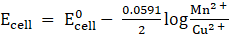→ Equation 2Substituting Equation 1 in equation 2, we get,∴ Ecell = 0.34 - ( - 2.37) -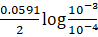= 2.71 -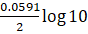= 2.71 - 0.02955∴ Ecell = 2.68 VThe e.m.f of the cell, Ecell is 2.68 Vii) Fe + 2H + → Fe2 + + H2 (n = 2)E0H+/H2 = 0 VE0Fe2 +/Fe = - 0.44 VE0cell = E0R - E0LE0cell = 0 - ( - 0.44) → Equation 1Using Nernst equation, we get,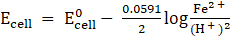→Equation 2Substituting Equation 1 in equation 2, we get,∴ Ecell = 0 - ( - 0.44) -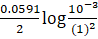= 0.44 -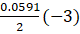= 0.44 - 0.0887∴ Ecell = 0.5287 VThe e.m.f of the cell, Ecell is 0.5287 Viii) Sn + 2H + → Sn2 + + H2 (n = 2)E0H+/H2 = 0 VE0Sn2 +/Sn = - 0.14VE0cell = E0R - E0LE0cell = 0 - ( - 0.14) → Equation 1Using Nernst equation, we get,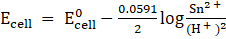→Equation 2Substituting Equation 1 in equation 2, we get,∴ Ecell = 0 - ( - 0.14) -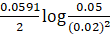= 0.14 -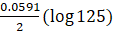= 0.14 - 0.0295×2.0969∴ Ecell = 0.08 VThe e.m.f of the cell, Ecell is 0.08 Viv) 2Br - + 2H + → Br2 + H2 (n = 2)E0H+/H2 = 0 VE0Br2/Br- = 1.08VE0cell = E0R - E0LE0cell = 0 - (1.08) → Equation 1Using Nernst equation, we get,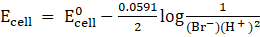→Equation 2Substituting Equation 1 in equation 2, we get,∴ Ecell = 0 - (1.08) -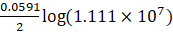= - 1.08 -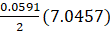= - 1.08 - 0.208∴ Ecell = 1.288 VThe e.m.f of the cell, Ecell is 1.288 VQuestion 6.In the button cells widely used in watches and other devices the following reaction takes place:Zn(s) + Ag2O(s) + H2O(l)→ Zn2+(aq) + 2Ag(s) + 2OH–(aq). Determine ΔrG0 and E0 for the reaction.Answer:Given - Zn → Zn2 + + 2e - , E0 = 0.76V (anode)Ag2O + H2O + 2e - →2Ag + 2OH - , E0 = 0.344V (cathode), n = 2ΔrG0 = ?E0cell = ?Zn is oxidized and Ag2O is reduced.Hence, the standard cell potential, E0cell is given as,E0cell = E0R - E0LE0cell = 0.344 + 0.76∴ E0cell = 1.104 VTo calculate the standard Gibb’s free energy, ∆rG0, we use,∆rG0 = - nE0F → Equation 1= - 2×96487×1.104 J= - 213043.296 J∴ ∆rG0 = - 2.13×105 JThe standard cell potential,E0cell is 1.104 V and thestandard Gibb’s free energy, ∆rG0 is- 2.13×105 JQuestion 7.Define conductivity and molar conductivity of the solution of an electrolyte. Discuss their variation with concentration.Answer:The conductivityof a solution is defined as the conductance of one unit volume of solution kept between two platinum electrodes with a unit area of cross-section and at a distance of unit length.The molar conductivityof the solution is defined as the conducting power of all the ions produced by one gram mole of an electrolyte in a solution. It is denoted by ∧m.The conductivityof a solution (both for strong and weak electrolytes) always decreases with the decrease in concentration of the electrolyte i.e., on dilution. This pattern is seen because the number of ions per unit volume that carry the current in the solution decreases on dilution. The molar conductivity of the solution increases with the decrease in concentration of the electrolyte. This is because both the number of ions as well as mobility of ions increase with dilution.Question 8.The conductivity of 0.20 M solution of KCl at 298 K is 0.0248 S cm–1. Calculate its molar conductivity.Answer:Given -Molarity, C = 0.20 MElectrolytic conductivity of a solution, κ = 0.0248 S cm–1Molar conductivity = ?Molar conductivity, ∧m =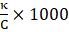S cm2 mol-1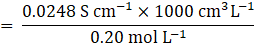∴ ∧m = 124 S cm2 mol-1Molar conductivity(∧m) of 0.20 M solution of KCl at 298 K is 124 Scm2 mol-1Question 9.The resistance of a conductivity cell containing 0.001M KCl solution at 298 K is 1500 Ω. What is the cell constant if the conductivity of 0.001M KCl solution at 298 K is 0.146 × 10–3 S cm–1.Answer:Given -Resistance of a conductivity cell, R = 1500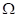Electrolytic conductivity of a solution, κ = 0.146 × 10–3 S cm–1Cell constant = ?Conductivity, κ =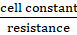Cell constant = κ × R= 0.146 × 10–3 S cm–1×1500Cell constant = 0.219 cm-1The cell constant of the cell containing 0.001M KCl solution at 298 K is 0.219 cm-1Question 10.The conductivity of sodium chloride at 298 K has been determined at different concentrations and the results are given below:Calculate Λm for all concentrations and draw a plot between Λm and c1/2. Find the value of Λ0m.Answer: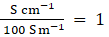(unit conversion factor)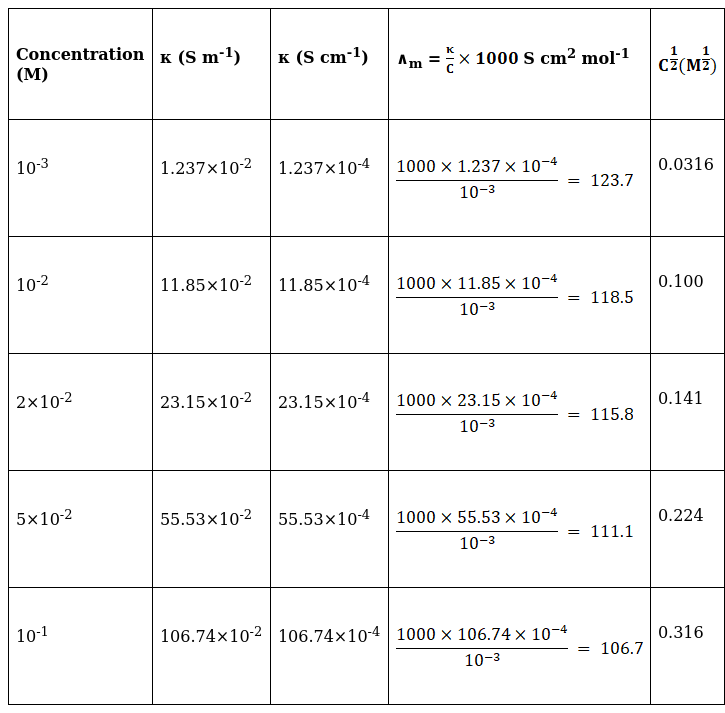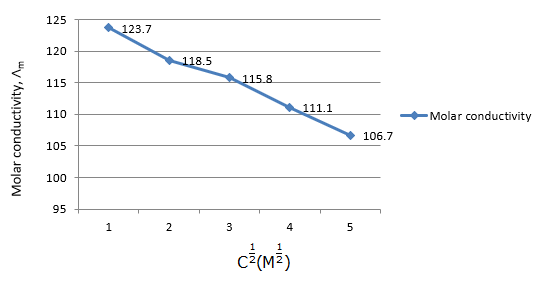∧0m= Intercept on the∧maxis = 124.0 S cm2 mol-1, which is obtained by extrapolation to zero concentration.Question 11.Conductivity of 0.00241 M acetic acid is 7.896 × 10–5 S cm–1. Calculate its molar conductivity. If ∧0m for acetic acid is 390.5 S cm2mol–1, what is its dissociation constant?Answer:Given -Molarity, C = 0.00241 MConductivity, κ = 7.896 × 10–5 S cm–1Molar conductivity, ∧m = ?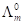for acetic acid = 390.5 S cm2mol–1Molar conductivity, ∧m =S cm2 mol-1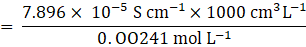∴ ∧m = 32.76 S cm2 mol-1To calculate the dissociation constant, Ka, we useKa =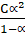→ Equation 1Here, we need to find the value of α (degree of dissociation), by the formula,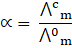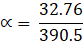∴ α = 8.4×10-2 ⇒ Equation 2→ Thus, substituting Equation 2 in Equation 1, we get,Ka ==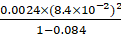∴ Ka = 1.86×10 - 5Themolar conductivity,∧mis32.76S cm2 mol-1 and thedissociation constant, Ka is1.86×10-5Question 12.How much charge is required for the following reductions:(i) 1 mol of Al3 + to Al?(ii) 1 mol of Cu2 + to Cu?(iii) 1 mol of MnO–4 to Mn2+ ?Answer:(i) The electrode reaction is given as,Al3 +(aq) + 3e - → Al(s)∴ The quantity of charge required for the reduction of 1 mol of Al3+ = 3F= 3×96487 C= 289461 C(ii) The electrode reaction is given as,Cu2 +(aq) + 2e - → Cu(s)∴ The quantity of charge required for the reduction of 1 mol of Cu2+ = 2F= 2×96487 C= 192974 C(iii) The electrode reaction is given as,MnO4→ Mn2 +i.e., Mn7 + + 5e - → Mn2 +∴ The quantity of charge required for the reduction of 1 mol of Mn7+ = 5F= 5×96487 C= 482435 CQuestion 13.How much electricity in terms of Faraday is required to produce(i) 20.0 g of Ca from molten CaCl2?(ii) 40.0 g of Al from molten Al2O3?Answer:(i) Ca2+ + 2e- → Ca⇒ Here, 1 mole of Ca, i.e., 40g of Ca requires = 2 F electricity (F if Faraday)∴ 20g of Ca requires =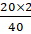= 1 F of electricityElectricity in terms of Faraday required to produce 20.0 g of Ca from molten CaCl2 is1 F of electricity.(ii) Al3 + + 3e - → Al⇒ 1 mole of Al, i.e., 27g of Al requires = 3 F electricity (F if Faraday)∴ 40.0 g of Al will require =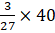= 4.44 F of electricityElectricity in terms of Faraday required to produce 40.0 g of Al from molten Al2O3 is 4.44 F of electricityQuestion 14.How much electricity is required in coulomb for the oxidation of(i) 1 mol of H2O to O2?(ii) 1 mol of FeO to Fe2O3?Answer:(i)The electrode reaction for 1 mole of H2O is given as,H2O → H2 +O2i.e., O2 - →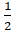O2 + 2e -∴ The quantity of electricity required = 2F= 2×96487 C= 192974 CThe quantity of electricity required in coulomb for the oxidation of 1 mol of H2O to O2 is 192974 C(ii) The electrode reaction for 1 mole of FeO isFeO +O2 →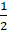Fe2O3i.e., Fe2 + → Fe3 + + e -∴ The quantity of electricity required = 1F= 1×96487 C= 96487 CThe quantity of electricity required in coulomb for the oxidation of 1 mol of FeO to Fe2O3 is 96487 CQuestion 15.A solution of Ni(NO3)2 is electrolysed between platinum electrodes using a current of 5 amperes for 20 minutes. What is mass of Ni deposited at the cathode?Given -Current = 5ATime - 20 minutesMass of Ni deposited = ?Answer:Quantity of electricity passed = 5 A × (20 × 60 sec)= 6000 C ⇒ Equation 1The electrode reaction is written as,Ni2 + + 2e → NiThus, the quantity of electricity required = 2F= 2×96487 C= 192974 C∵ 192974 C of electricity deposits 1 mole of Ni, which is 58.7 g ⇒ Equation 2Thus, equating equations 1 and 2, we get,192974 C of electricity deposits = 58.7 g6000 C of electricity will deposit =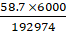= 1.825g of NiThe mass of Ni deposited at the cathode is 1.825g of NiQuestion 16.Three electrolytic cells A,B,C containing solutions of ZnSO4, AgNO3 and CuSO4, respectively are connected in series. A steady current of 1.5 amperes was passed through them until 1.45 g of silver deposited at the cathode of cell B. How long did the current flow? What mass of copper and zinc were deposited?Given -I = 1.5 AW = 1.45 g of Agt = ?n = 1Answer:Equivalent weight is Ag, EAg =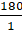= 180Equivalent weight is Cu, ECu =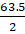= 31.75Equivalent weight is Zn, EZn=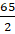= 32.5Using Faraday’s second law of electrolysis, to find the mass of Cu and Zn, we use Equation 1,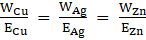→ Equation 1⇒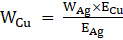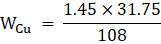∴ WCu = 0.426 g⇒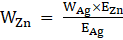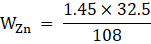∴ WZn = 0.436 gTo find the time of current flow, using Faraday’s first law of electrolysis we get,M = Z ×I ×t ⇒ Equation 2∵ Z =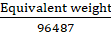, Equation 2 becomes,M =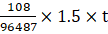t =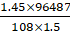t = 864 seconds.The time of current flow, t = 864 seconds, the mass of Cu is 0.426 g and mass of Zn is 0.436 gQuestion 17.Using the standard electrode potentials given in Table 3.1, predict if the reaction between the following is feasible:(i) Fe3 + (aq) and I–(aq)(ii) Ag + (aq) and Cu(s)(iii) Fe3 + (aq) and Br– (aq)(iv) Ag(s) and Fe3 + (aq)(v) Br2 (aq) and Fe2 + (aq).Answer:(i) The electrode reaction is written as,2Fe3 + + 2I - → 2Fe2 + + I2E0cell =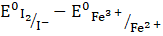= 0.54V - 0.77V∴ E0cell = - 0.23 VIt is not feasible, as E0cell is negative, ∴ ∆G0 is positive.(ii) The electrode reaction is written as,2Ag +(aq) + Cu(s)→ Cu2 +(aq) + Ag(s)E0cell =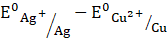= + 0.80V - 0.34V∴ E0cell = 0.46VIt is feasible, as E0cell is positive, ∴ ∆G0 is negative.(iii) The electrode reaction is written as,2Fe3 +(aq) + 2Br -(aq)→ 2Fe2 +(aq) + Br2E0cell =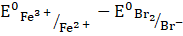= 0.77V - 1.09V∴ E0cell = - 0.32 VIt is not feasible, as E0cell is negative, ∴ ∆G0 is positive.(iv) The electrode reaction is written as,Ag(s) + Fe3 +(aq) → Fe2 +(aq) + Ag +(aq)E0cell =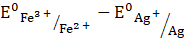= 0.77V - 0.80V∴ E0cell = - 0.03It is not feasible, as E0cell is negative, ∴ ∆G0 is positive.(v) The electrode reaction is written as,Br2 + 2Fe2 +(aq) → 2Br -(aq) + 2Fe3 +(aq)E0cell =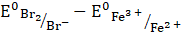= 1.09V - 0.77V∴ E0cell = 0.32 VIt is feasible, as E0cell is positive, ∴ ∆G0 is negative.Question 18.Predict the products of electrolysis in each of the following:(i) An aqueous solution of AgNO3 with silver electrodes.(ii) An aqueous solution of AgNO3 with platinum electrodes.(iii) A dilute solution of H2SO4 with platinum electrodes.(iv) An aqueous solution of CuCl2 with platinum electrodes.Answer:Given -All the ions are in aqueous state.(i) Reaction in solution:AgNO3(s) + aq → Ag + + NO3 -H2O ó H + + OH -At cathode:Ag +(aq) + e - →Ag(s)Ag + ions have lower discharge potential than H + ions. Hence, Ag + ions get deposited as Ag in preference to H + ions.At anode:Ag(s)→ Ag +(aq) + e -As Ag anode is attacked by NO3 - ions, Ag of the anode will dissolve to form Ag + ions in the aqueous solution.(ii) Reaction in solution:AgNO3(s) + aq → Ag + + NO3 -H2O óH + + OH -At cathode:2Ag +(aq) + 2e - →2Ag(s)Ag + ions have lower discharge potential than H + ions. Hence, Ag + ions get deposited as Ag in preference to H + ions.At anode:2OH -(aq) → O2(g) + 2H +(aq) + 4e -As anode is not attackable, out of OH - and NO3 - ions, OH - having lower discharge potential, will be discharged in preference to NO3 - ions. These then decompose to give out O2.(iii) Reaction in solution:H2SO4(aq) → 2H +(aq) + SO42 -(aq)At cathode:2H +(aq) + 2e - →H2(g)At anode:2OH -(aq) → O2(g) + 2H +(aq) + 4e -∴H2 gas is evolved at cathode and O2(g) is evolved at anode.(iv) Reaction in solution:CuCl2(s) + aq → Cu2 +(aq) + Cl -(aq)H2O óH + + OH -At cathode:Cu2 +(aq) + 2e - →Cu(g)At anode:2Cl -(aq) - 2e - → Cl2(g)∴Cu will be deposited at cathode and Cl2 gas will be liberated at anode.

PDF FILE TO YOUR EMAIL IMMEDIATELY PURCHASE NOTES & PAPER SOLUTION. @ Rs. 50/- each (GST extra)

HINDI ENTIRE PAPER SOLUTION

MARATHI PAPER SOLUTION

SSC MATHS I PAPER SOLUTION

SSC MATHS II PAPER SOLUTION

SSC SCIENCE I PAPER SOLUTION

SSC SCIENCE II PAPER SOLUTION

SSC ENGLISH PAPER SOLUTION

SSC & HSC ENGLISH WRITING SKILL

HSC ACCOUNTS NOTES

HSC OCM NOTES

HSC ECONOMICS NOTES

HSC SECRETARIAL PRACTICE NOTES

2019 Board Paper Solution

HSC ENGLISH SET A 2019 21st February, 2019

HSC ENGLISH SET B 2019 21st February, 2019

HSC ENGLISH SET C 2019 21st February, 2019

HSC ENGLISH SET D 2019 21st February, 2019

SECRETARIAL PRACTICE (S.P) 2019 25th February, 2019

HSC XII PHYSICS 2019 25th February, 2019

CHEMISTRY XII HSC SOLUTION 27th, February, 2019

OCM PAPER SOLUTION 2019 27th, February, 2019

HSC MATHS PAPER SOLUTION COMMERCE, 2nd March, 2019

HSC MATHS PAPER SOLUTION SCIENCE 2nd, March, 2019

SSC ENGLISH STD 10 5TH MARCH, 2019.

HSC XII ACCOUNTS 2019 6th March, 2019

HSC XII BIOLOGY 2019 6TH March, 2019

HSC XII ECONOMICS 9Th March 2019

SSC Maths I March 2019 Solution 10th Standard11th, March, 2019

SSC MATHS II MARCH 2019 SOLUTION 10TH STD.13th March, 2019

SSC SCIENCE I MARCH 2019 SOLUTION 10TH STD. 15th March, 2019.

SSC SCIENCE II MARCH 2019 SOLUTION 10TH STD. 18th March, 2019.

SSC SOCIAL SCIENCE I MARCH 2019 SOLUTION20th March, 2019

SSC SOCIAL SCIENCE II MARCH 2019 SOLUTION, 22nd March, 2019

XII CBSE - BOARD - MARCH - 2019 ENGLISH - QP + SOLUTIONS, 2nd March, 2019

HSC Maharashtra Board Papers 2020

(Std 12th English Medium)

HSC ECONOMICS MARCH 2020

HSC OCM MARCH 2020

HSC ACCOUNTS MARCH 2020

HSC S.P. MARCH 2020

HSC ENGLISH MARCH 2020

HSC HINDI MARCH 2020

HSC MARATHI MARCH 2020

HSC MATHS MARCH 2020

SSC Maharashtra Board Papers 2020

(Std 10th English Medium)

English MARCH 2020

HindI MARCH 2020

Hindi (Composite) MARCH 2020

Marathi MARCH 2020

Mathematics (Paper 1) MARCH 2020

Mathematics (Paper 2) MARCH 2020

Sanskrit MARCH 2020

Sanskrit (Composite) MARCH 2020

Science (Paper 1) MARCH 2020

Science (Paper 2)

MUST REMEMBER THINGS on the day of Exam

Are you prepared? for English Grammar in Board Exam.

Paper Presentation In Board Exam

How to Score Good Marks in SSC Board Exams

Tips To Score More Than 90% Marks In 12th Board Exam

How to write English exams?

How to prepare for board exam when less time is left

How to memorise what you learn for board exam

No. 1 Simple Hack, you can try out, in preparing for Board Exam

How to Study for CBSE Class 10 Board Exams Subject Wise Tips?

JEE Main 2020 Registration Process – Exam Pattern & Important Dates

NEET UG 2020 Registration Process Exam Pattern & Important Dates

How can One Prepare for two Competitive Exams at the same time?

8 Proven Tips to Handle Anxiety before Exams!